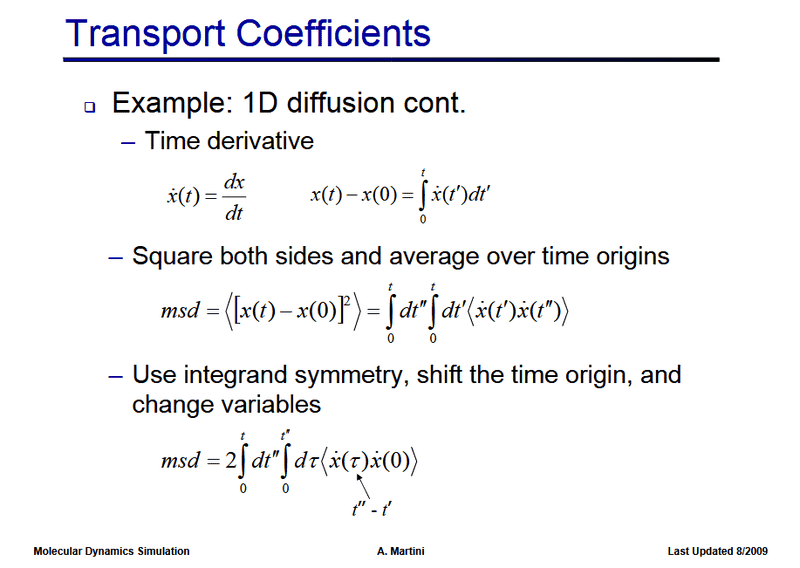Equivalence of msd and velocity autocorrelation

Greetings,

I am searching for an explanation that connects the mean squared displacement in an ensemble of particles to the velocity autocorrelation functions. I know that they are connected since both of them can be used to calculate the diffusion constant. But I do not find a mathematical derivation. Does anyone have some link or material here?

Best,
SL

I have found the following sheet:First question: What does it mean to average "over time origins"?
In my simulation, I calculate the msd via averaging over particle trajectories, not different time origins.

Second question: What exactly happens in the last step? I can't replicate it, if I rewrite t' in terms of TAU and t''.
From the second line, I write ## t'=t'' - \tau ##.
I get ##\int_0^t \, dt'' (- \int_{t''}^{t''-t} \, d\tau <v(t''-\tau)v(t'')>) = \int_0^t \, dt'' \int_{t''-t}^{t''} \, d\tau <v(t''-\tau)v(t'')>##

But that's not the same, is it?

Attachments

Andy Resnick
I am searching for an explanation that connects the mean squared displacement in an ensemble of particles to the velocity autocorrelation functions.

I'm not sure how to best answer- there are lots of online materials that work through it, but let's just start with the Fourier-transformed position x(ω) = v(ω)/iω. Then, <[Δx(t)]2> = <v2>t2. Note, <v2 is not the same as the velocity autocorrelation function: for a Maxwell-Boltzmann distribution at temperature T,

<v(t)v(t')> = (v02-T/m)e-γ(t+t')+T/m e-γ|t-t'|

and
<[x(t)-x(t')]2>=##<[\int_{t'}^t \, dt_1 v(t_1) ]^2>##

Does that help?

I'm not sure how to best answer- there are lots of online materials that work through it, but let's just start with the Fourier-transformed position x(ω) = v(ω)/iω. Then, <[Δx(t)]2> = <v2>t2. Note, <v2 is not the same as the velocity autocorrelation function: for a Maxwell-Boltzmann distribution at temperature T,

<v(t)v(t')> = (v02-T/m)e-γ(t+t')+T/m e-γ|t-t'|

and
<[x(t)-x(t')]2>=##<[\int_{t'}^t \, dt_1 v(t_1) ]^2>##

Does that help?

Thank you, but not really... I would like to understand it without Fourier or Maxwell Boltzmann, as it should be a more trivial connection, see my calculation above.